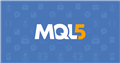# close all position in average profit

Hello. I am looking for how can I write a code that will simply allow me to close all positions incurred with an average profit of \$ 10 for example, for all sets of negative or positive trades. THANK YOU

***Documentation on MQL5: Constants, Enumerations and Structures / Environment State / Symbol Properties
• www.mql5.com
Symbol Properties - Environment State - Constants, Enumerations and Structures - MQL5 Reference - Reference on algorithmic/automated trading language for MetaTrader 5

Hannah Tshilumba:

Hello. I am looking for how can I write a code that will simply allow me to close all positions incurred with an average profit of \$ 10 for example, for all sets of negative or positive trades. THANK YOU

***

Hello, this should do the trick

```
if (AccountEquity()<AccountBalance()-Risk)

{
int count=0;
for(int i=0; i < OrdersTotal(); ){
if(OrderSelect(i, SELECT_BY_POS)
&& OrderType()   == OrderType()
&& OrderSymbol() == Symbol()
&& OrderClose(OrderTicket(), OrderLots(), OrderClosePrice(), 10)
){ RefreshRates(); // Update OrderClosePrice for next select.
i=0;            // Reprocess all orders, possible race condition.
++count;
}
else ++i;
}
return count;
}

if (AccountEquity()>AccountBalance()+Reward)

{
int count=0;
for(int i=0; i < OrdersTotal(); ){
if(OrderSelect(i, SELECT_BY_POS)
&& OrderType()   == OrderType()
&& OrderSymbol() == Symbol()
&& OrderClose(OrderTicket(), OrderLots(), OrderClosePrice(), 10)
){ RefreshRates(); // Update OrderClosePrice for next select.
i=0;            // Reprocess all orders, possible race condition.
++count;
}
else ++i;
}
return count;```

Of course, you need to enter what is risk and what is reward

Hannah Tshilumba:

Hello. I am looking for how can I write a code that will simply allow me to close all positions incurred with an average profit of \$ 10 for example, for all sets of negative or positive trades. THANK YOU

***

```double AvProfit=10;
if(TotalProfit>=PositionsTotal()*AvProfit){
return;
}
}```

Quite easy, hope this helps.

Luis Garcia #:

Hello, this should do the trick

Of course, you need to enter what is risk and what is reward

This is MQL5, not 4.

Hannah Tshilumba:

Hello. I am looking for how can I write a code that will simply allow me to close all positions incurred with an average profit of \$ 10 for example, for all sets of negative or positive trades. THANK YOU

***

```double balance   = AccountInfoDouble (ACCOUNT_BALANCE);
double equity    = AccountInfoDouble (ACCOUNT_EQUITY);
double AvgProfit = m_position.Profit()/PositionsTotal();
if(equity >= balance + 10 && equity >= balance + AvgProfit)
{
CloseAllPositions();
return;
}```

CodeFx #:
`double AvgProfit = m_position.Profit()/PositionsTotal();`

how to get it m_position.Profit() ???

```double balance   = AccountInfoDouble (ACCOUNT_BALANCE);